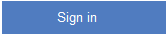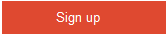# What is an equivalence partition (also known as an equivalence class)?

+1 vote
47 viewsposted May 10Equivalence partitioning is a type of Black box testing techniques. In Equivalence testing, the whole input range is divided into a set of equivalence classes.

Example: A program should be tested with input range from 1 to 1000.

Here the Equivalent classes are:
1) less than 1 ( <1 ) ; 2) between 1 and 1000 ; 3) greater than 1000 ( >1000 )

Any value can be taken from each equivalence class and can be used for testinganswer May 15answer May 12